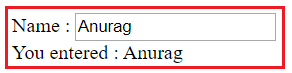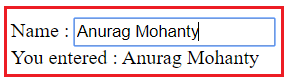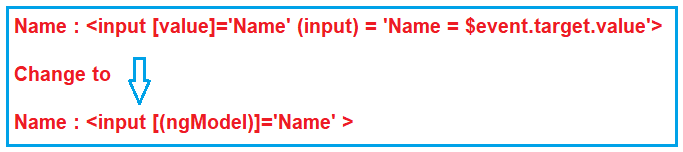# Angular Two Way Binding

## Angular Two Way Data Binding with Examples

In this article, I am going to discuss the Angular Two Way Data Binding with examples. Please read our previous article where we discussed Angular Event Binding in Detail. At the end of this article, you will understand the following pointers in detail which are related to Angular Two Way data binding.

1. What is Angular Two Way Binding?
2. Example to understand Angular Two Way Data Binding
3. Using the input event of the input control
4. Two Way Data binding using ngModel Directive
##### What is Angular Two Way Binding?

The most popular and widely used data binding mechanism in Angular Application is two-way data binding. The two-way data binding is basically used in the input type filed or any form element where the user type or provide any value or change any control value on the one side and on the other side, the same automatically updated into the component variables and vice-versa is also true.The two-way data binding in Angular is actually a combination of Property Binding and Event Binding. The Syntax is given below:

<input [value] = ‘data 1’ (input) = ‘data = \$event.target.value’>

##### Two-Way Binding using ngModel Directive:

You can also implement the two-way data binding in Angular Application using the ngModel directive. The ngModel directive combines the square brackets of property binding with the parentheses of event binding in a single notation. The syntax to use ngModel for two-way data binding is given below.

<input [(ngModel)] = ‘data’>

If you are getting confused at the moment, then don’t worry, we will try to explain two-way data binding using both the approaches.

##### Example to understand Angular Two Way Data Binding:

Let’s directly start with an example. First, modify the app.component.ts file as shown below.

```import { Component } from '@angular/core';
@Component({
selector: 'app-root',
template: `<div>
Name : <input [value]='Name'>
<br>
You entered : {{Name}}
</div>`
})
export class AppComponent {
Name: string = 'Anurag';
}
```

From the above code, we need to understand two things.

1. <input [value]=’Name’>: it binds the component class “Name” property to the value property of the input element.
2. You entered: {{Name}}: The Interpolation displays the value we have in the “Name” property of the Component class on the web page.

So, when you browse the application, you should see the value Anurag in both the places as expected as shown in the below image.At this moment if you change the value in the textbox, then that changed value is not going to be reflected in the browser (i.e. in the second line i.e. after you entered string). There are two different mechanisms that you can use to make them reflected (i.e. two-way data binding). Let us discuss one by one.

##### Using the input event of the input control:

One way to achieve two-way data binding is by binding to the input event of the input control. So, modify the app.component.ts file as shown below which and then we will understand the code.

```import { Component } from '@angular/core';
@Component({
selector: 'app-root',
template: `<div>
Name : <input [value]='Name' (input) = 'Name = \$event.target.value'>
<br>
You entered : {{Name}}
</div>`
})
export class AppComponent {
Name: string = 'Anurag';
}
```

Let’s understand the above code

1. [value]=’Name’: This is property binding and it flows the data from the component class Name property to element property i.e. value property of the input element.
2. (input)=’Name = \$event.target.value’: This is event binding and it flows the data in the opposite direction i.e. from the element to the component class property i.e. “Name”
3. \$event: It is provided by angular event binding and it contains the event data. To retrieve the values from the input element, you need to use it as – \$event.target.value.
4. Name = \$event.target.value: This expression updates the value in the Name property of the component class
5. You entered : {{name}}: This interpolation expression will then display the updated value on the web page.

I hope now you understand the code present in the app.component.ts file. At this point, open the browser and start typing in the textbox. As you start typing that changed value will be reflected immediately on the page as shown in the below image.##### Two Way Data binding using ngModel Directive:

To simplify the two-way data binding, the angular framework has provided one directive called the ngModel directive. With the ngModel directive, you can change to existing code as shown below.With the above changes in place in the app.component.ts file, at this point, if you run the application, then you will get the following error.This is because the ngModel directive is available in the system module called FormsModule. If you want to use the ngModel directive, then in your root module that is AppModule, you will have to import the FormsModule first.

##### Steps to use ngModel Directive:

Here are the steps to import FormsModule into our AppModule

1. Open app.module.ts file
2. Include the following import statement in it
import { FormsModule } from ‘@angular/forms’;
3. Also, include FormsModule in the ‘imports’ array of @NgModule
imports: [BrowserModule, FormsModule]

With the above changes in place, the complete code of App.Module.ts is as follows.

```import { NgModule } from '@angular/core';
import { AppRoutingModule } from './app-routing.module';
import { AppComponent } from './app.component';

import { FormsModule } from '@angular/forms';

@NgModule({
declarations: [
AppComponent
],

imports: [
BrowserModule,
AppRoutingModule,
FormsModule
],

providers: [],
bootstrap: [AppComponent]
})

export class AppModule { }
```

The complete codes of app.component.ts file as follows:

```import { Component } from '@angular/core';
@Component({
selector: 'app-root',
template: `<div>
Name : <input [(ngModel)]='Name'>
<br>
You entered : {{Name}}
</div>`
})
export class AppComponent {
Name: string = 'Anurag';
}
```

With the above changes, now reload the web page and you should see everything is working as expected.

### 6 thoughts on “Angular Two Way Binding”

1.Thank you for the ngModel

2.Fantastic explanation to compare angular io

3.Getting below error when I use the code you mentioned above for two way data binding without ngModel directive, could you please tell me what went wrong

Error: src/app/app.component.ts:5:76 – error TS2531: Object is possibly ‘null’.

Name :

1.Put (input) = \$any(\$event.target).value

4.As I read on the internet the difference \$any makes is that it doesn’t look at the type of the element

5.Excellent, Awesome way of elaboration